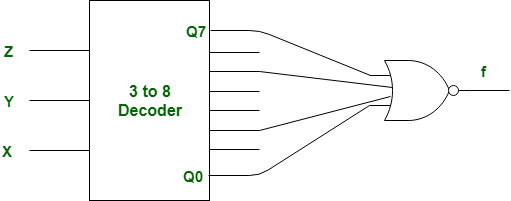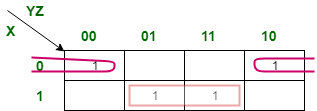Open in App
Not now

# GATE | Gate IT 2008 | Question 9

• Difficulty Level : Medium
• Last Updated : 20 Aug, 2019

What Boolean function does the circuit below realize ?(A) xz+x’z’
(B) xz’+x’z
(C) x’y’+yz
(D) xy+y’z’

Explanation: There 0, 2, 5, 7 are enable input in given decoder.

Therefore, given K-Map should be as following:So, output of above K-Map is,

```= xz + x'z'
= (x⊙z)
= x (ex-nor) z ```

But, there given gate is NOR instead of OR, therefore above output will be negatated.
So, output function f is,

```f = (xz + x'z')'
= x'z + xz'
= (x⊕z)
= x (xor) z ```

So, option (B) is correct.

This explanation is contributed by Deep Shah.

Quiz of this Question

My Personal Notes arrow_drop_up
Related Articles